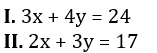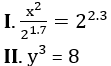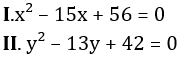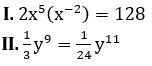Dear Aspirants,

Quantitative Aptitude Quiz For SBI PO/Clerk Prelims

Numerical Ability or Quantitative Aptitude Section has given heebie-jeebies to the aspirants when they appear for a banking examination. As the level of every other section is only getting complex and convoluted, there is no doubt that this section, too, makes your blood run cold. The questions asked in this section are calculative and very time-consuming. But once dealt with proper strategy, speed, and accuracy, this section can get you the maximum marks in the examination. Following is the Quantitative Aptitude quiz to help you practice with the best of latest pattern questions.

Directions (1-5): In each of these questions, two equations (I) and (II) are given. You have to solve both the equations and give answer

Q1.if x > y
if x ≥ y
if x < y
if x ≤ y
if x = y or no relation can be established between x and y.

Q2.if x > y
if x ≥ y
if x < y
if x ≤ y
if x = y or no relation can be established between x and y.

Q3.if x > y
if x ≥ y
if x < y
if x ≤ y
if x = y or no relation can be established between x and y.

Q4.if x > y
if x ≥ y
if x < y
if x ≤ y
if x = y or no relation can be established between x and y.

Q5.if x > y
if x ≥ y
if x < y
if x ≤ y
if x = y or no relation can be established between x and y.

Directions (6-10): What approximate value will come in place of question mark (?) in following questions?

Q6. 133.99 + 1333.98 + 13.98 = ?

1452
1482
1575
1650
1730

Q7.224
254
242
340
324

Q8.1900
1980
1890
2010
2100

Q9.6
4
2
8
10

Q10.25
20
15
10
18

Directions (11-15): In each of these questions two equations are given. You have to solve these equations and give answer.

Q11.if x < y
if x > y
if x = y
if x ≥ y
if x ≤ y

Q12.if x < y
if x > y
if x = y
if x ≥ y
if x ≤ y

Q13.if x < y
if x > y
if x = y
if x ≥ y
if x ≤ y

Q14.if x < y
if x > y
if x = y
if x ≥ y
if x ≤ y

Q15.if x < y
if x > y
if x = y
if x ≥ y
if x ≤ y

You May also like to Read: